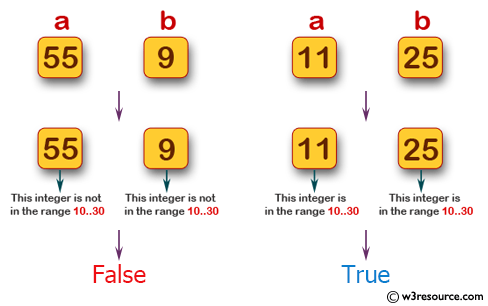﻿ Swift Basic Programming Exercise: Return true if either of two given integers is in the range 10..30 inclusive - w3resource# Swift Basic Programming Exercise: Return true if either of two given integers is in the range 10..30 inclusive

## Swift Basic Programming: Exercise-12 with Solution

Write a Swift program that return true if either of two given integers is in the range 10..30 inclusive.

Pictorial Presentation:Sample Solution:

Swift Code:

``````func in1030(a: Int, b: Int) -> Bool {
if a >= 10 && a <= 30
{
return true
}
else if b >= 10 && b <= 30
{
return true
}
else
{
return false
}
}
print(in1030(a: 15, b: 40))
print(in1030(a: 55, b: 9))
print(in1030(a: 11, b: 25))
```
```

Sample Output:

```true
false
true
```

Swift Programming Code Editor:

Improve this sample solution and post your code through Disqus

What is the difficulty level of this exercise?

﻿